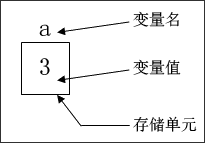# C语言常量与变量

## 常量

• 整型常量：12、0、-3；
• 实型常量：4.6、-1.23；
• 字符常量：‘a’、‘b’。

#define 标识符 常量

【例3-1】符号常量的使用。
```#include<stdio.h>
#define PRICE 30
int main(){
int num,total;
num=10;
total=num* PRICE;
printf("total=%d",total);
return 0;
}

• 用标识符代表一个常量，称为符号常量。
• 符号常量与变量不同，它的值在其作用域内不能改变，也不能再被赋值。
• 使用符号常量的好处是：含义清楚；能做到“一改全改”。

## 变量• 允许在一个类型说明符后，定义多个相同类型的变量。各变量名之间用逗号间隔。类型说明符与变量名之间至少用一个空格间隔。
• 最后一个变量名之后必须以“;”号结尾。
• 变量定义必须放在变量使用之前。一般放在函数体的开头部分。

1. int num,total;
2. double price = 123.123;
3. char a = 'a', abc;

#### 变量的赋值

类型说明符 变量1= 值1, 变量2= 值2, ……;

1. int a=3;
2. int b,c=5;
3. float x=3.2,y=3.0,z=0.75;
4. char ch1='K',ch2='P';

```
```

1. #include<stdio.h>
2. int main(void){
3. int a=3,b,c=5;
4. b=a+c;
5. printf("a=%d,b=%d,c=%d\n",a,b,c);
6. return 0;
7. }

(10)
76.9%

(3)
23.1%